### Contact

New Features
• RegisterSSI has enjoyed great success over the years in the development and publishing of statistical software and is proud to announce the release of LISREL 10.1.

In an effort to meet the growing demands of our LISREL 8 and 9 user community, SSI has developed LISREL 10, which is on the cutting edge of current technology. The program has been tested extensively on the Microsoft Windows platform with Windows 7 and Windows 10 operating systems.

## Background

Structural equation modeling (SEM) was introduced initially as a way of analyzing a covariance or correlation matrix. Typically, one would read this matrix into LISREL and estimate the model by maximum likelihood. If raw data was available without missing values, one could also use PRELIS first to estimate an asymptotic covariance matrix to obtain robust estimates of standard errors and chi-squares.

Modern structural equation modeling is based on raw data. With LISREL 10, if raw data is available in a LISREL data system file or in a text file, one can read the data into LISREL and formulate the model using either SIMPLIS syntax or LISREL syntax.

LISREL 10 contains fixes to all bugs reported by users of LISREL 9. The new LISREL features are summarized next.

## Multiple group analyses using a single data file

In practice, many multivariate data sets are observations from several groups. Examples of these groups are genders, languages, political parties, countries, faculties, colleges, schools, etc.

LISREL may be used to fit multiple group structural equation models to multiple group data. Traditional statistical methods such as Maximum Likelihood (ML), Robust Maximum Likelihood (RML), Weighted Least Squares (WLS), Diagonally Weighted Least Squares (DWLS), Generalized Least Squares (GLS) and Un-weighted Least Squares (ULS) are available for complete multiple group data while the Full Information Maximum Likelihood (FIML) method is available for incomplete multiple group data.

In previous versions of LISREL, the user was required to create separate data files for each group. Suppose that the groups to be analyzed consisted of data collected in eight countries, the implication is that eight datasets must to be created in order to fit a multiple group structural equation model.

A new feature implemented in LISREL 10 allows researchers to use a single dataset that contains a group variable that can be defined by

• Using the Data menu when a LISREL system file (.lsf) is opened
• By inserting the line \$GROUPS=<group variable name> anywhere in the syntax file.

Consider the dataset efficacy_4countries.lsf shown below. There are 4 countries and portion of the data from countries 2 and 3 are shown below.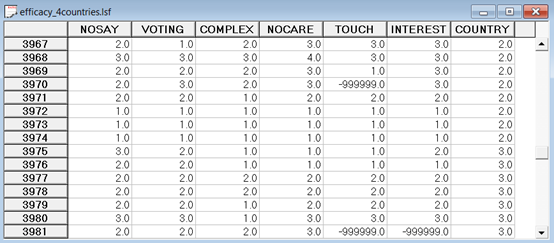To use this dataset in a multiple group analysis, use the Data menu from the main menu bar and select the Group Variable… option (see below)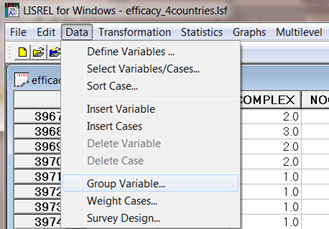Select COUNTRY from the list of variables and when done click the OK button.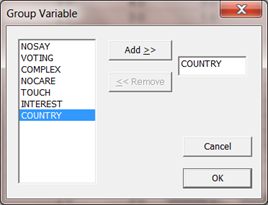The LISREL Examples folder contains a sub-folder named MGROUPS that contains examples for the following statistical procedures: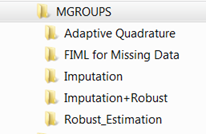For a detailed example, see the Assessment of Invariance, (Section 2 in the “Additional Topics Guide.pdf”) that can be accessed via the Help option on the main menu bar: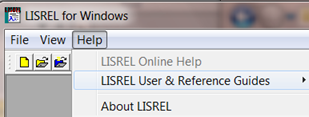## Models for grouped- and discrete-tie survival data

Models for grouped-time survival data are useful for analysis of failure time data when subjects are measured repeatedly at fixed intervals in terms of the occurrence of some event, or when determination of the exact time of the event is only known within grouped intervals of time. Additionally, it is often the case that subjects are observed nested within clusters (i.e., schools, firms, clinics), or are repeatedly measured in terms of recurrent events. In this case, use of grouped-time models that assume independence of observations is problematic since observations from the same cluster or subject are usually correlated.

For data that are clustered and/or repeated, models including random effects provide a convenient way of accounting for association in correlated survival data.

Several authors have noted the relationship between ordinal regression models (using complementary log-log and logistic link functions) and survival analysis models for grouped and discrete time. In LISREL 10 a generalization of an ordinal random-effects regression model to handle correlated grouped-time survival data is implemented. This model accommodates multivariate normally-distributed random effects, and additionally, allows for a general form for model covariates.

Assuming a proportional or partial proportional, hazards or odds model, a maximum marginal likelihood solution is implemented using multi-dimensional quadrature to numerically integrate over the distribution of random-effects. The reference guide “Survival Models for grouped data.pdf” contains examples and references and is accessible via the online Help menu.

## Models for ordinal outcomes and the proportional odds versus non-proportional odds assumption

The term "ordinal" is applied to variables where the response measure of interest is measured in a series of ordered categories. Examples of such variables include Likert scales and psychiatric ratings of severity. Nominal and ordinal outcome models can be seen as generalizations of the binary outcome model. The ordinal model becomes important when the outcome variable is not dichotomous, or not truly continuous. If an ordinal outcome is analyzed within a continuous model, such a model can yield predicted values outside the range of the ordinal variable. As with binary data, some transformation or link function becomes necessary to prevent this from happening. The continuous model can also yield correlated residuals and regressors when applied to ordinal outcomes because the continuous model does not take the ceiling and floor effects of the ordinal outcome into account. This can then result in biased estimates of regression coefficients, and is most critical when the ordinal variable in question is highly skewed.

Extensive work on the development of methods for the analysis of ordinal response data has been undertaken by numerous researchers. These developments have focused on the extension of methods for dichotomous variables to ordinal response data, and have been mainly in terms of logistic and probit regression models. The proportional odds model is a common choice for the analysis of ordinal data. In LISREL 10, it is possible to fit both proportional and non-proportional odds models to verify the proportional odds assumption using a chi-square difference test.  The reference guide “Models for proportional and non-proportional odds.pdf” contains examples and references and is accessible via the online Help menu.

## P-value for C1 statistic under normality

Modern structural equation modeling is based on raw data. With LISREL 10, if raw data is available in a LISREL data system file or in a text file, one can read the data into LISREL and formulate the model using either SIMPLIS syntax or LISREL syntax. It is no longer necessary to estimate an asymptotic covariance matrix with PRELIS and read this into LISREL. The estimation of the asymptotic covariance matrix and the model is now done in LISREL. One can also use the EM or MCMC multiple imputation methods in LISREL to fit a model to the imputed data.

## LISREL and PRELIS functionality

Satorra & Bentler (1978) states that the asymptotic distribution of chi-square C1 is that of a linear combination of chi-squares with 1 degree of freedom, where the coefficients of the linear combination are the d (d = the degree of freedom) non-zero eigenvalues of UW_NNT, where U is defined in Satorra & Bentler (1978) and also in equation (45) in the document "New Features in LISREL 9" (available under "LISREL User & Reference Guides") and W_NNT is an estimate of the asymptotic covariance matrix of the variances and covariance of the observed variables under non-normality, usually referred to as the ACM matrix.

LISREL 10 estimates the d eigenvalues and the p-value of the linear combination. Essentially, this makes the other chi-squares C2, C3, C4 and C5 less important, since they are based on approximations of the distribution using only the mean and variance of the eigenvalues.

## Data conversion using stat/transfer

The data import/export software has been upgraded from Stat/Transfer Version 13 to the most recently released Version 14.

Selection of new features available Stat/Transfer Version 14

Version 14 has added support for the following formats:

• Stata 15/MP
• BayesiaLab (Write Only)
• JSON-Stat (Read Only)Version 14 has larger limits for:
•
• Excel Files > 4 GB
• SAS files > 32K variables
• Stata Files > 32K variables
• dBASE > 2GB

## FIML for ordinal and continuous variables

LISREL 10 supports Structural Equation Modeling for a mixture of ordinal and continuous variables for simple random samples and complex survey data.

The LISREL implementation allows for the use of design weights to fit SEM models to a mixture of continuous and ordinal manifest variables with or without missing values with optional specification of stratum and/or cluster variables. It also deals with the issue of robust standard error estimation and the adjustment of the chi-square goodness of fit statistic.

This method is based on adaptive quadrature and a user can specify any one of the following four link functions:

• Logit
• Probit
• Complementary Log-log
• Log-Log

Examples to illustrate this feature are given in the folders \orfimlex and \ls9ex.

## Three-level Multilevel Generalized Linear Models

Cluster or multi-stage samples designs are frequently used for populations with an inherent hierarchical structure. Ignoring the hierarchical structure of data has serious implications. The use of alternatives such as aggregation and disaggregation of information to another level can induce an increase in co-linearity among predictors and large or biased standard errors for the estimates.

The collection of models called Generalized Linear Models (GLIMs) have become important, and practical, statistical tools. The basic idea of GLIMs is an adaption of standard regression to quite different kinds of data. The variables may be dichotomous, ordinal (as with a 5-point Likert scale), counts (number of arrest records), or nominal. The motivation is to tailor the regression relationship connecting the outcome to relevant independent variables so that it is appropriate to the properties of the dependent variable. The statistical theory and methods for fitting Generalized Linear Models (GLIMs) to survey data was implemented in LISREL 8.8.

Researchers from the social and economic sciences are often applying these methods to multilevel data and consequently, inappropriate results are obtained. The LISREL statistical module for the analysis of multilevel data allows for design weights. Two estimation methods, MAP (maximization of the posterior distribution) and QUAD (adaptive quadrature) for fitting generalized linear models to multilevel data are available. The LISREL Multilevel Generalized Linear models module (MGLIM) allows for a wide variety of sampling distributions and link functions.

The LISREL 10 MGLIM module also include zero-inflated Poisson and zero-inflated Negative-Binomial models and prints results for unit-specific and population-average estimates of the fixed effects.

Examples in the folder \mglimex illustrate these features.

## Four and Five-level Multilevel Models for continuous outcome variables

Social science research often entails the analysis of data with a hierarchical structure. A frequently cited example of multilevel data is a dataset containing measurements on children nested within schools, with schools nested within education departments.

The need for statistical models that take account of the sampling scheme is well recognized and it has been shown that the analysis of survey data under the assumption of a simple random sampling scheme may give rise to misleading results.

Multilevel models are particularly useful in the modeling of data from complex surveys. Cluster or multi-stage samples designs are frequently used for populations with an inherent hierarchical structure. Ignoring the hierarchical structure of data has serious implications. The use of alternatives such as aggregation and disaggregation of information to another level can induce an increase in co-linearity among predictors and large or biased standard errors for the estimates. In order to address concerns regarding the appropriate analyses of survey data, the LISREL multilevel module for continuous data now also handles up to five levels and features an option for users to include design weights on levels 1, 2 , 3, 4 or 5 of the hierarchy.

Examples are given in the \mlevelex folder.

## Filename extensions

All LISREL syntax files have extension .lis (since LISREL 9, previously .ls8), all PRELIS syntax files have extension .prl (since LISREL 9, previously .pr2). The LISREL spreadsheet has been renamed LISREL data system file and has extension .lsf (since LISREL 9, previously .psf).

To ensure backwards compatibility, users can still run previously created syntax files using a .psf file, but to open an existing .psf file using the graphical user’s interface, the user has to rename it to .lsf.

## MVABOOK examples

These examples are based on a new book: "Multivariate Analysis with LISREL" authored by Karl G Jöreskog, Ulf H. Olsson & Fan Y. Wallentin (2017). The book is published by Springer-Verlag and is available both as a hardcover book and as an e-book at

This book can be used by Master and PhD students and researchers in the economic, social, behavioral, and many other sciences that need to have a basic understanding of multivariate statistical theory and methods for their analysis of multivariate data. It can also be used as a text book for courses on multivariate statistical analysis. All examples are listed in the Table of Contents. All the syntax and data files for these examples are distributed with LISREL 10.1 and are located in

• LISREL Examples\MVABOOK\CHAPTER1
• LISREL Examples\MVABOOK\CHAPTER2
• LISREL Examples\MVABOOK\CHAPTER3
• LISREL Examples\MVABOOK\CHAPTER4
• LISREL Examples\MVABOOK\CHAPTER5
• LISREL Examples\MVABOOK\CHAPTER6
• LISREL Examples\MVABOOK\CHAPTER7
• LISREL Examples\MVABOOK\CHAPTER8
• LISREL Examples\MVABOOK\CHAPTER9
• LISREL Examples\MVABOOK\CHAPTER10

## Running LISREL in batch mode

Any of the LISREL programs can be run into batch mode by using a .bat file with the following script:

"c:\program files (x86)\LISREL10\MLISREL64_10"

where

Program name = LISREL, PRELIS, MULTILEV, MAPGLIM or SURVEYGLIM

Example:

Syntax File = "c:\LISREL Examples\ls9ex\npv1a.spl"

Output File = "c:\LISREL Examples\ls9ex\npv1a.out"

Examples of batch files (RunLISREL.bat and RunSIMPLIS.bat) are given in the \ls9ex folder. These batch files will run all the LISREL and SIMPLIS syntax files in this folder.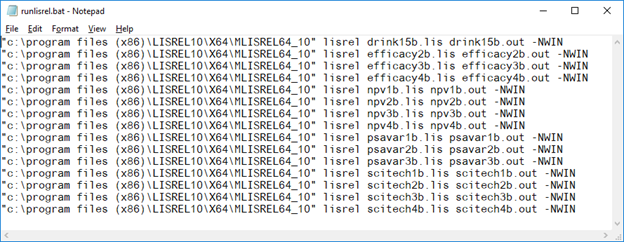## Documentation

Program documentation is available as PDFs via the Help menu.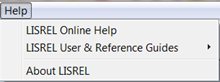• New features in LISREL
• Graphical User’s Interface
• PRELIS Examples Guide
• LISREL Examples Guide
• Multilevel Modeling Guide
• Complex Survey Sampling
• Generalized Linear Modeling Guide
• Multilevel Generalized Linear Modeling Guide
• Models for Proportional and Non-proportional Odds
• Survival Models for Grouped Data
• LISREL Syntax Guide
• SIMPLIS Syntax Guide
• PRELIS Syntax Guide

Documentation of the LISREL graphical user’s interface is also available as an online Help file. The Help file has features that simplify navigation across topics: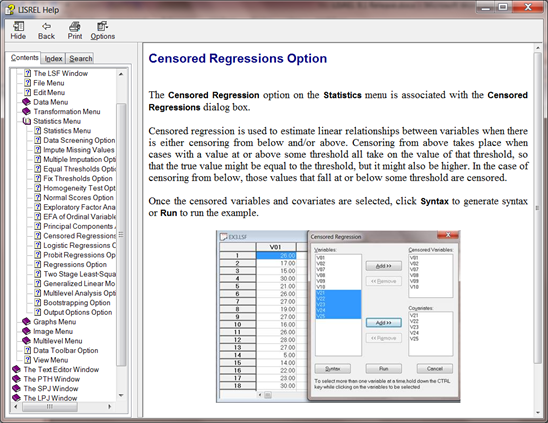The Complex Survey Sampling Guide includes structural equation modeling (SEM) for continuous variables and SEM for a mixture of ordinal and continuous variables. LISREL uses full information maximum likelihood under complex survey data with data missing at random.

The Additional Topics Guide includes sections on assessment of invariance, multiple imputation, multilevel structural equation modeling and multilevel non-linear regression.

## Examples

The syntax and data files for the examples are installed in the folder C:\LISREL Examples\.

A selection of examples, illustrating some of the new features is given below.

## Analysis of ordinal data using quadrature (\ls9ex)

Efficacy3a.spl: Model 2 Estimated by FIML
Raw Data from file EFFICACY.LSF
Latent Variables Efficacy Respons
Relationships
NOSAY - NOCARE = Efficacy
NOCARE - INTEREST = Respons
Path Diagram
End of Problem

Path Diagram Representation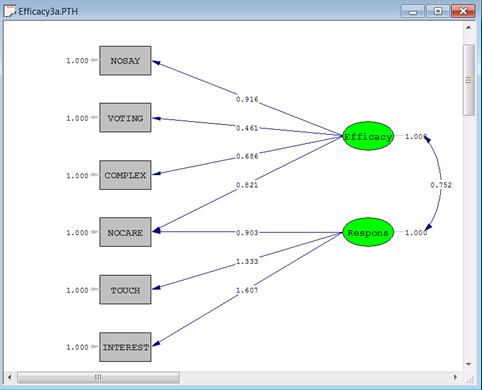Path Diagram (Standardized Solution)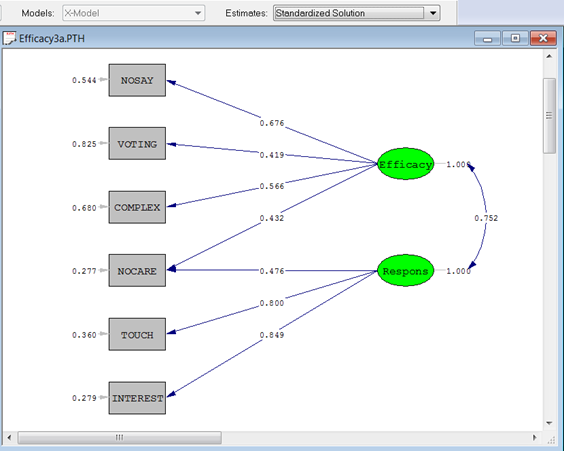Portion of output file

The last part of the output file is shown below.

For the moment we note the value of the deviance statistic −2 ln L = 19858.06. Since there is no value of −2 ln L for a saturated model, it is impossible to say whether this is large or small in some absolute sense. The deviance statistic can therefore only be used to compare different models for the same data.

To illustrate, the difference between the deviance statistic for this model and the deviance statistic for a model with one latent variable (Efficacy2a.spl) is 19934.57-19858.06 =76.51, which suggests that the two-dimensional model fits the data much better than the uni-dimensional model.

The output also gives estimates of the thresholds, their standard errors and z-values. The thresholds are parameters of the model but are seldom useful in analysis of a single sample.